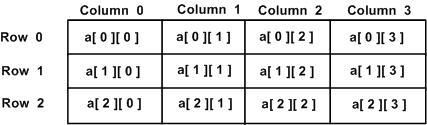# Pascal - Multi-dimensional Array

Pascal programming language allows multidimensional arrays. Here is the general form of a multidimensional array declaration −

```type
array-identifier = array [index-type1, index-type2, ...] of element-type;
var
a1, a2, ... : array-identifier;
```

For example, the following declaration creates a three dimensional 5 . 10 . 4 integer array −

```var
threedim: array[1..5, 1..10, 1..4] of integer;
```

## Two-Dimensional Arrays

The simplest form of the multidimensional array is the two-dimensional array. A two-dimensional array is, in essence, a list of one-dimensional arrays. To declare a two-dimensional integer array of size x, y you would write something as follows −

```var
arrayName: array[1..x, 1..y] of element-type;
```

Where element-type can be any valid Pascal data type and arrayName will be a valid Pascal identifier. A two-dimensional array can be visualized as a table, which will have x number of rows and y number of columns. A 2-dimensional array that contains three rows and four columns can be shown as below −Thus, every element in array a is identified by an element name of the form a[ i ][ j ], where a is the name of the array, and i and j are the subscripts that uniquely identify each element in a.

## Initializing Two-Dimensional Arrays

Multidimensional arrays, like one-dimensional array, are initialized by through assignment, either by specifying a particular subscript or using a for-do loop.

For example,

```var
a: array [0..3, 0..3] of integer;
i, j : integer;

begin
for i:= 0 to 3 do
for j:= 0 to 3 do
a[i,j]:= i * j;
...
end;
```

## Accessing Two-Dimensional Array Elements

An element in 2-dimensional array is accessed by using the subscripts, i.e., row index and column index of the array. For example −

```var
val: integer;
val := a[2, 3];
```

The above statement will take 4th element from the 3rd row of the array. You can verify it in the above diagram. Let us check below program where we have used nested loop to handle a two-dimensional array −

```program ex2dimarray;
var
a: array [0..3, 0..3] of integer;
i,j : integer;

begin
for i:=0 to 3 do
for j:=0 to 3 do
a[i,j]:= i * j;

for i:=0 to 3 do
begin
for j:=0 to 3 do
write(a[i,j]:2,' ');
writeln;
end;
end.
```

When the above code is compiled and executed, it produces the following result −

```0 0 0 0
0 1 2 3
0 2 4 6
0 3 6 9
```

As explained above, you can have arrays with any number of dimensions, although it is likely that most of the arrays you create will be of one or two dimensions.

pascal_arrays.htm